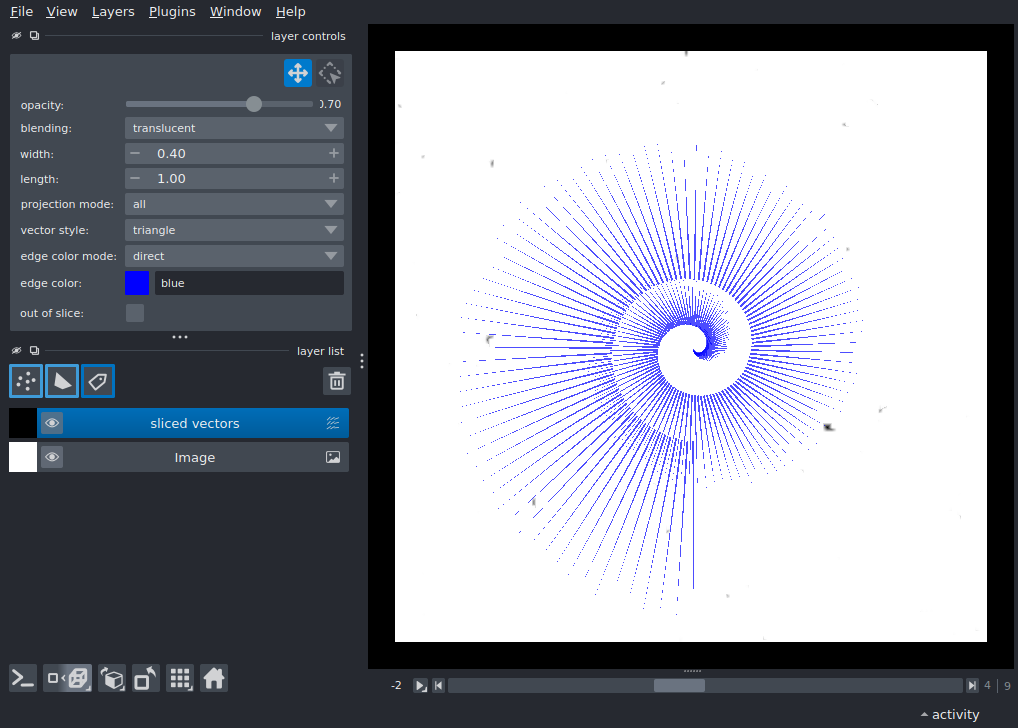# nD vectors¶

Display two vectors layers ontop of a 4-D image layer. One of the vectors layers is 3D and “sliced” with a different set of vectors appearing on different 3D slices. Another is 2D and “broadcast” with the same vectors apprearing on each slice.

Tags: visualization-nD```import numpy as np
from skimage import data
import napari

blobs = np.stack(
[
data.binary_blobs(
length=128, blob_size_fraction=0.05, n_dim=3, volume_fraction=f
)
for f in np.linspace(0.05, 0.5, 10)
],
axis=0,
)
viewer = napari.view_image(blobs.astype(float))

# sample vector coord-like data
n = 200
pos = np.zeros((n, 2, 2), dtype=np.float32)
phi_space = np.linspace(0, 4 * np.pi, n)

# assign x-y position
pos[:, 0, 0] = radius_space * np.cos(phi_space) + 64
pos[:, 0, 1] = radius_space * np.sin(phi_space) + 64

# assign x-y projection
pos[:, 1, 0] = 2 * radius_space * np.cos(phi_space)
pos[:, 1, 1] = 2 * radius_space * np.sin(phi_space)

planes = np.round(np.linspace(0, 128, n)).astype(int)
planes = np.concatenate(
(planes.reshape((n, 1, 1)), np.zeros((n, 1, 1))), axis=1
)
vectors = np.concatenate((planes, pos), axis=2)